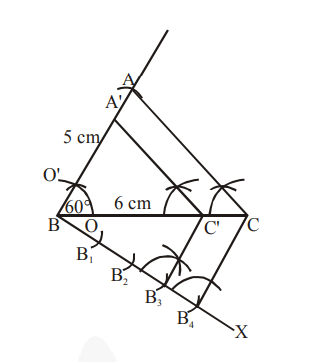# Draw a triangle ABC with side BC = 6 cm,

Question:

Draw a triangle $\mathrm{ABC}$ with side $\mathrm{BC}=6 \mathrm{~cm}, \mathrm{AB}=5 \mathrm{~cm}$ and $\angle \mathrm{ABC}=60^{\circ}$.

Then construct a triangle whose sides are $\frac{\mathbf{3}}{\mathbf{4}}$ of the corresponding sides of the triangle $\mathrm{ABC}$.

Solution:

Steps of construction :

1. Draw a $\triangle \mathrm{ABC}$ with side $\mathrm{BC}=6 \mathrm{~cm}, \mathrm{AB}=5 \mathrm{~cm}$ and $\angle \mathrm{ABC}=60^{\circ}$.

2. Draw a ray $\mathrm{BX}$ making an acute angle with $\mathrm{BC}$ on the opposite side of vertex $\mathrm{A}$.

3. Mark 4 points (as 4 is greater in 3 and 4$), B_{1}, B_{2}, B_{3}, B_{4}$, on line segment $B X$ such that $\mathrm{BB}_{1}=\mathrm{B}_{1} \mathrm{~B}_{2}=\mathrm{B}_{2} \mathrm{~B}_{3}=\mathrm{B}_{3} \mathrm{~B}_{4}$

4. Join $\mathrm{B}_{4} \mathrm{C}$ and draw a line through $\mathrm{B}_{3}$, parallel to $\mathrm{B}_{4} \mathrm{C}$ intersecting $\mathrm{BC}$ at $\mathrm{C}^{\prime}$.

5. Draw a line through $C^{\prime}$ parallel to $A C$ intersecting $A B$ at $A^{\prime} . \Delta A^{\prime} B C^{\prime}$ is the required triangle.